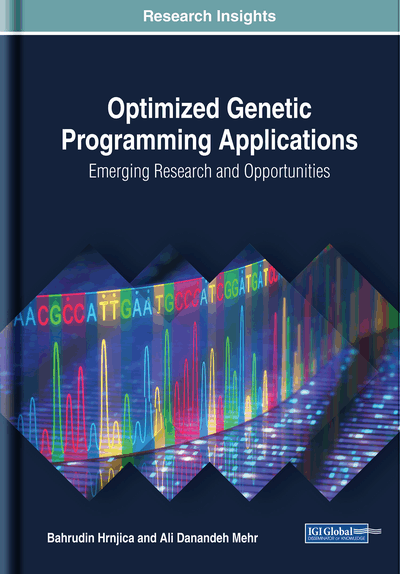# Genetic Programming Applications in Solving Engineering Problems

DOI: 10.4018/978-1-5225-6005-0.ch006
Available
\$37.50
No Current Special Offers

## Abstract

This chapter explains how to use genetic programming to solve various kinds of problems in different engineering fields. Here, three applications, each of which relevant to a distinct engineering field, are explained. First, the chapter starts with the GP application in mechanical engineering. The application analyzes the use of GP in modelling impact toughness of welded joint components. The experimental results of impact toughness represent the input data to build GP models of each welded joint component individually. The second part of the chapter shows how two recent versions of GPdotNET can be satisfactorily used for a binary classification-prediction problem in civil engineering. This application puts forward a new classification-forecasting model, namely binary GP for teleconnection studies between oceanic and heavily local hydrologic variables. Finally, the third application demonstrates how GP could be applied to solve a time series forecasting problem in the field of electrical engineering.
Chapter Preview
Top

## Application 1: The Application Of Genetic Programming In Modelling Impact Toughness Of Welded Joint Components

### Introduction to Impact Toughness Testing

Bend testing with impact force effect on notched specimens can provide an explanation of the behavior of materials under obstructed strain, i.e. the spatial stress state. Determination of work needed for fracture under specified test conditions is commonly used for the current quality control and homogeneity of the material, as well as its processing. This testing procedure can determine the tendency to brittle fracture or a tendency towards increased brittleness during exploitation (Viehring, 2002). The impact energy experimental results obtained by testing the general structural steel S235 JG were used for the application of genetic programming in modeling impact toughness of welded joint components. V-notched specimens were tested at different temperatures on instrumented Charpy pendulum. This examination enabled separation of the total impact energy on the components, crack initiation energy and crack propagation energy. The experimental results of impact toughness represent the input data for the development of mathematical models of each welded joint component. Mathematical models of impact toughness were built using genetic programming, GP. Statistical analysis and comparison for GP models with the correspondent regression models was performed. Mathematical models obtained using GP provide a good basis for a quality and reliable planning of experimental research, with the aim of obtaining the relevant data in the entire process of determining fracture mechanics parameters (Hrnjica, 2014).

Impact testing of specimens of the base material (BM), weld metal (WM) and the heat affected zone (HAZ), was performed according to standard EN 10045-1 (EN-10045-1:1990, 1990) and ASTM E23-02 (ASTM-Standard-E23, 2002), on specimens whose geometry and layout are given in Figure 1, in order to determine the overall impact energy.

During bend impact loading, fracture energy is defined as an integral value. Such defined fracture energy does not allow the separation of the material resistance from the initiation and crack growth. In order to achieve this, impact force and time need to be recorded continuously during the testing, which is enabled with the instrumentation of the pendulum. Scheme of the modern instrumented pendulum is given in Figure 2.

In Figure 2, it is shown the instrumentation of pendulum which includes connection of force gauge, which is installed in the pendulum hammer, fracture time detector and strain gauge via amplifier with the oscilloscope. As the impact-caused specimen fracture is short-term instance (0.5 – 12 ms), the role of the oscilloscope is to make registered signals visible. The oscilloscope is then connected to a computer for processing received signals during the measurement.

Monitoring the changes of force and time makes it possible to learn more about whether the employed work for fracture pattern is a consequence of low-value force action for a longer time or short-term high-value force action, which is essential for the evaluation of material behavior. Examination of notched specimens on instrumented pendulum enabled monitoring of force changes in time, i.e. creating force-time diagram (See Figure 3).

Thus, the force - time diagram, Figure 3, determines magnitude of the following forces:

When the steep drop of force coincides with the maximum force, then. Forceis defined as a cross-section of steep drop of force - time curve and an oscillating smooth curve. Energy illustrated in the diagram can be calculated by integrating the force – time, which graphically present the numeric value of area under the curve. On this basis, we have:

(1) where F(t) is impact force, v(t) is pendulum rate during fracture, and t is time of fracture.

In addition to the total energy, it is possible to calculate which part of the energy is used for crack initiation, and which part for the crack propagation. In this regard, it is possible to use more than one method that can separate total impact energyto the crack initiation energy, and the crack propagation energy. The methods are based on the fact thatis not dependent on notch radius, but that the radius affects the crack propagation energy.

The optimum procedure for determining crack propagation energy is calculation on the “fatigue crack”. The essence of this procedure is to induce fatigue crack in the V-notched specimens. Such specimen is then fractured on the Charpy pendulum, and the used fracture energy is at the same time the crack propagation energy Ep. If the value of the total impact energy of the tested material is known, the part of the energy required for crack initiation,, can be calculated (Islamovic, 2006).

(2)

Determination of crack initiation energy and crack propagation energy with this method is conducted on one sample (as opposed to other methods), which provides greater accuracy. Figure 3 shows the marked areasandwhich exactly match the mentioned energy.

## Complete Chapter List

Search this Book:
Reset